# Convolutional Networks

Unreasonable Effectiveness of ConvNets on Object Recognition

A short-course on convolutional neural networks. The focus of this course is to familiarize students with the key ideas that underpin convolutional neural networks (for object recognition). The material is divided into two parts. Part 1 covers the beginnings of neural networks and tries to make a case for the importance of understanding basic techniques, such as line fitting, i.e., linear regression, in order to truly appreciate neural netowrks. Part 2 covers neural networks, including convolutional neural networks. Part 2 also lists important advances in convolutional neural networks from 2012 to the present day.

# Forward

Convolutional Neural Networks (ConvNets) have found tremendous success in many computer vision tasks, including object recognition. In a sense the so-called deep learning revolution, which has transformed the AI landscape, started with the October 2012 victory of AlexNet on the ImageNet Large Scale Visual Recognition Challenge. This short course tells the story of convolutional networks from their single-unit neural networks beginnings to their current success. Looking through the lens of object recognition, we will identify the key ideas and the scientific and technical developments that underpin convolutional neural networks. We will conclude this course with a brief survey of the recent trends in deep learning architectures for object recognition. This course is appropriate for senior undergraduate or graduate students. No prior knowledge in deep learning is assumed.

# Notes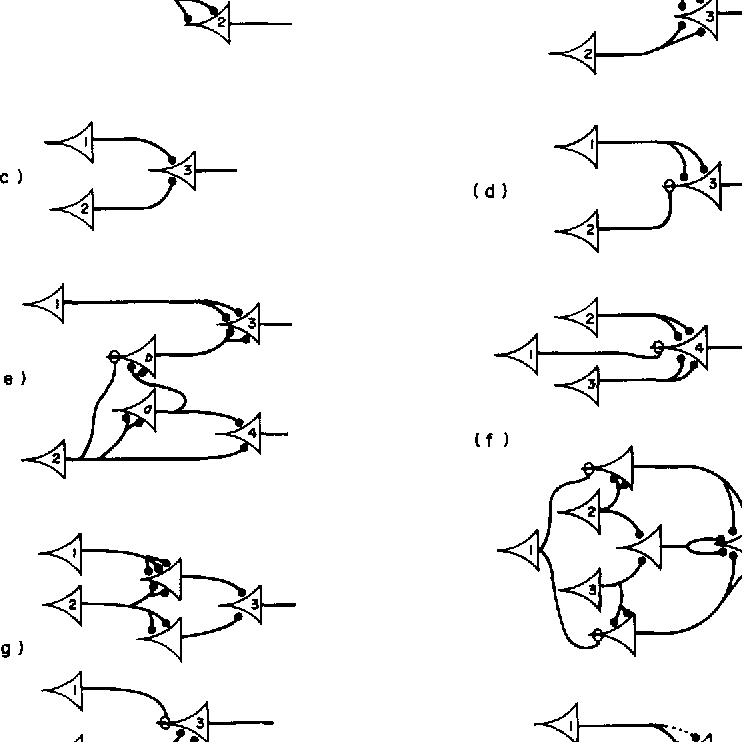##### A brief history of Neural Networks
• Computational models of Neurons
• Pre-deep learning
• Imagenet 2012
• Takeaways
• What
• How
• Why now?
• Impact
• Ethical and social implications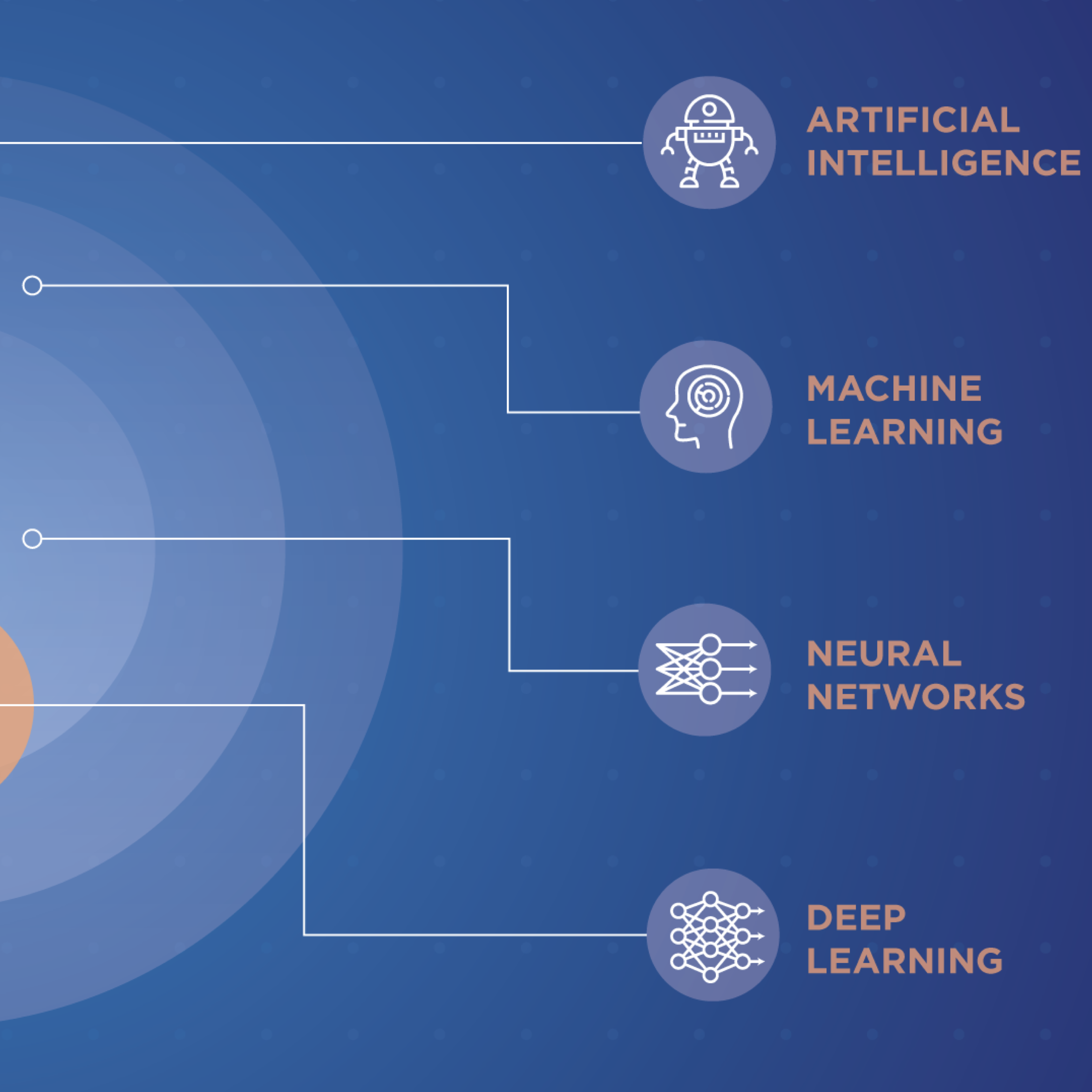##### Machine Learning
• What is Machine Learning (ML)
• ML and deep learning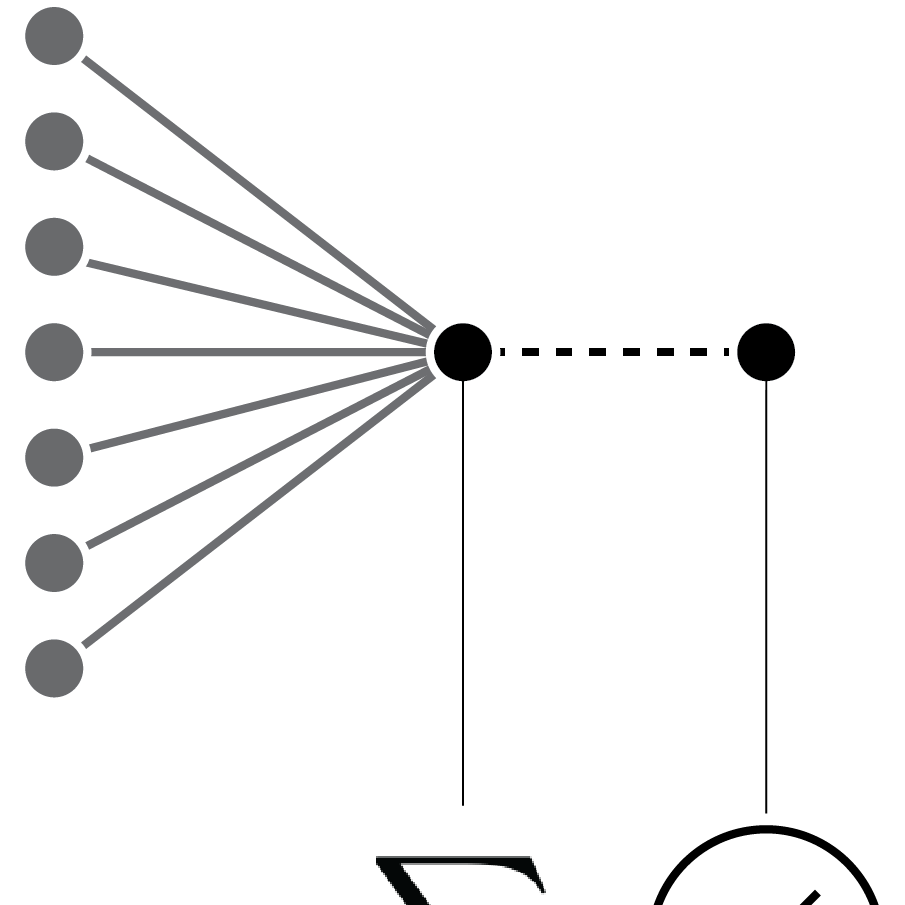##### Linear Regression
• Linear regression
• Model fitting through minimizing loss
• Dealing with non-linearities
• Model complexity and regularization
• Connection to Neural Networks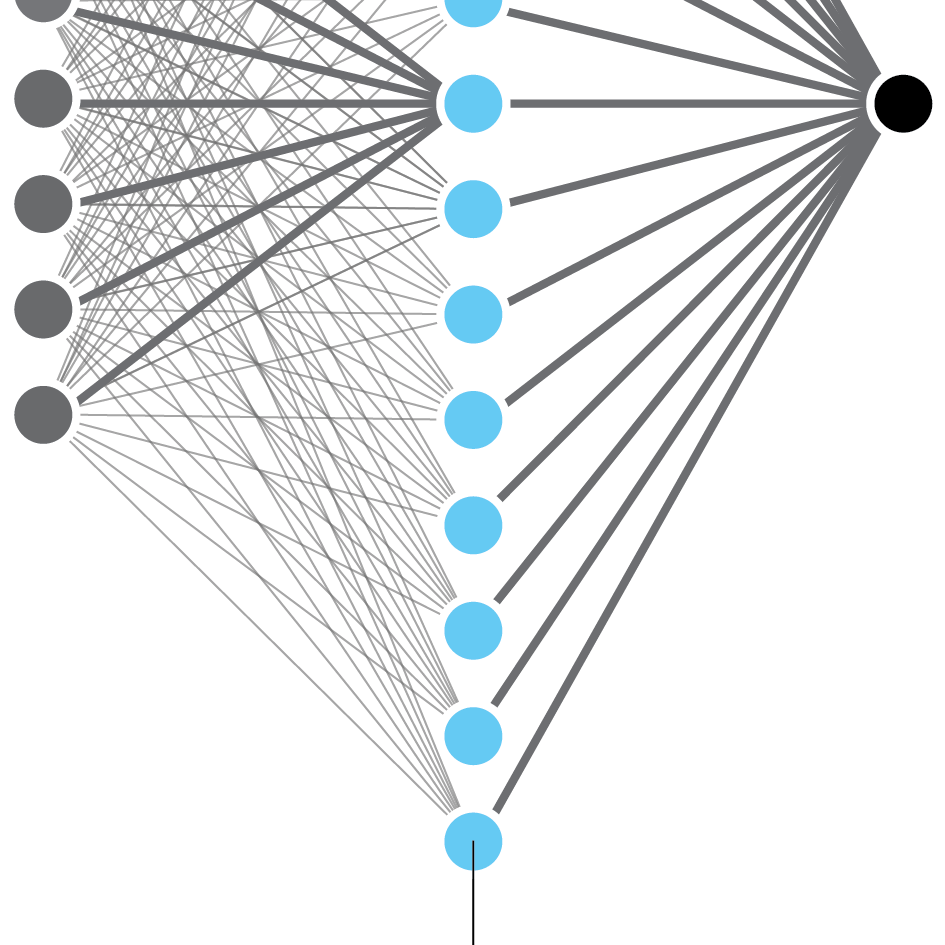##### Neural Networks
• Feed-forward neural networks
• Activation functions
• Gradient based learning in neural networks
• Backpropagation
• Layered view of neural networks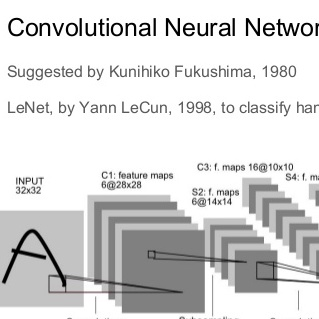##### Convolutional Networks
• Convolutional Networks
• Convolution
• Pooling layers
• Dilated convolutions
• Common architecture